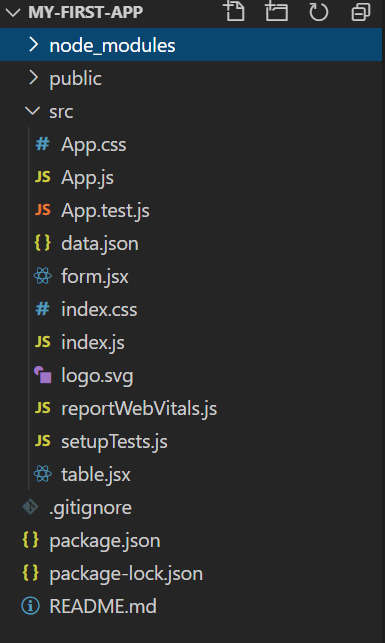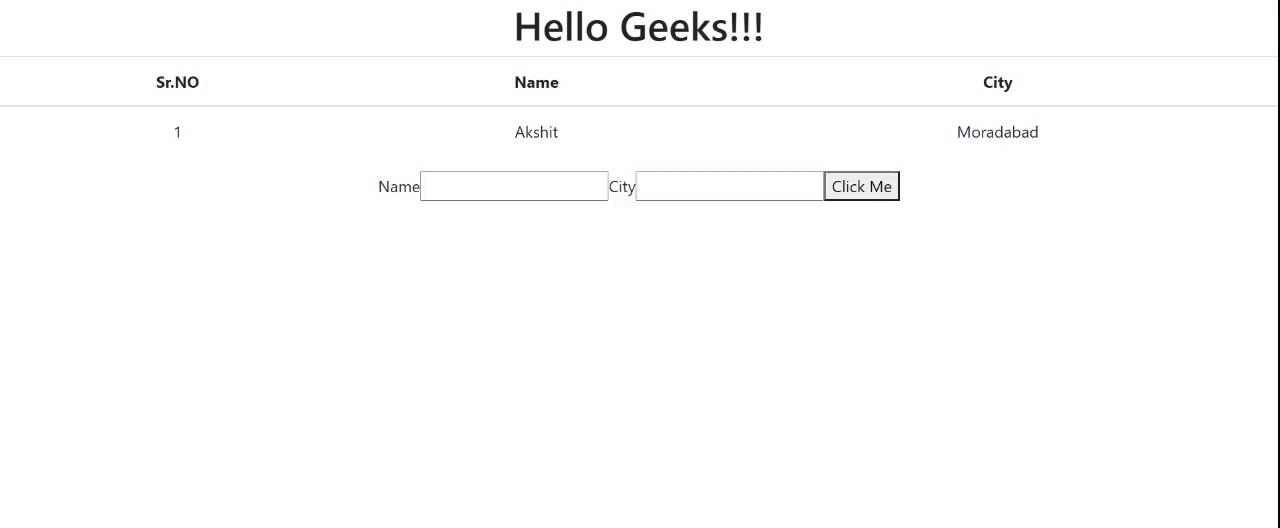# How to pass data into table from a form using React Components

ReactJS is a front-end library used to build UI components. In this article, we will learn to pass data into a table from a form using React Components. This will be done using two React components named Table and form. We will enter data into a form, which will be displayed in the table on ‘submit’.

Creating React Application And Installing Module:

Step 1: Create a React application using the following command.

`npx create-react-app `

Step 2: After creating your project folder(i.e. my-first-app), move to it by using the following command.

`cd my-first-app`

Project Structure: It will look like this.Step 3: Create a dummy JSON file; that initially contains the following one object and save it as data.json

`[ {"id":1,"name":"Akshit","city":"Moradabad"} ]`

Implementation: Now write down the following code in respective files.

## table.jsx

 `import React, { useState } from ``'react'``; ` ` `  `function` `StudentForm(props) { ` `  ``const [name, setName] = useState(``''``); ` `  ``const [city, setCity] = useState(``''``); ` ` `  `  ``const changeName = (event) => { ` `    ``setName(event.target.value); ` `  ``}; ` ` `  `  ``const changeCity = (event) => { ` `    ``setCity(event.target.value); ` `  ``}; ` ` `  `  ``const transferValue = (event) => { ` `    ``event.preventDefault(); ` `    ``const val = { ` `      ``name, ` `      ``city, ` `    ``}; ` `    ``props.func(val); ` `    ``clearState(); ` `  ``}; ` ` `  `  ``const clearState = () => { ` `    ``setName(``''``); ` `    ``setCity(``''``); ` `  ``}; ` ` `  `  ``return` `( ` `    ``
` `      `` ` `      `` ` `      `` ` `      `` ` `      `` ` `    ``
` `  ``); ` `} ` ` `  `export ``default` `StudentForm;`

## form.jsx

 `import React, { useState } from ``'react'``; ` `import StudentForm from ``'./form'``; ` `import jsonData from ``'./data.json'``; ` ` `  `function` `TableData() { ` `  ``const [studentData, setStudentData] = useState(jsonData); ` ` `  `  ``const tableRows = studentData.map((info) => { ` `    ``return` `( ` `      ``
{info.id}{info.name}{info.city}
` `        `` ` `        `` ` `        `` ` `      `` ` `    ``); ` `  ``}); ` ` `  `  ``const addRows = (data) => { ` `    ``const totalStudents = studentData.length; ` `    ``data.id = totalStudents + 1; ` `    ``const updatedStudentData = [...studentData]; ` `    ``updatedStudentData.push(data); ` `    ``setStudentData(updatedStudentData); ` `  ``}; ` ` `  `  ``return` `( ` `    ``
` `      `` ` `        ``
Sr.NONameCity
` `          `` ` `            `` ` `            `` ` `            `` ` `          `` ` `        `` ` `        ``{tableRows} ` `      `` ` `      `` ` `    `` ` `  ``); ` `} ` ` `  `export ``default` `TableData;`

## App.js

 `import TableData from ``'./form'``; ` ` `  `function` `App() { ` `  ``return` `( ` `    ``
` `      ``

Hello Geeks!!!

` `      `` ` `    ``
` `  ``); ` `} ` ` `  `export ``default` `App; `

Step to Run Application: Run the application using the following command from the root directory of the project.

`npm start`

Output: Now open your browser and go to http://localhost:3000/, you will see the following output:Whether you're preparing for your first job interview or aiming to upskill in this ever-evolving tech landscape, GeeksforGeeks Courses are your key to success. We provide top-quality content at affordable prices, all geared towards accelerating your growth in a time-bound manner. Join the millions we've already empowered, and we're here to do the same for you. Don't miss out - check it out now!

Previous
Next